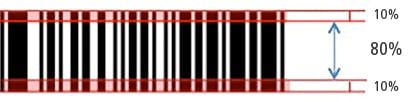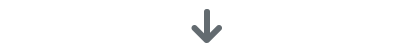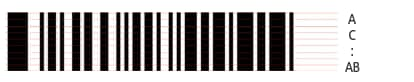# ISO/IEC 15416 Verification MethodWithin the center area that makes up 80% of the barcode (excluding the top and bottom 10%),10 scans worth of verification data are obtained every 8%.The grade of each scan is judged.The range of grades is A, B, C, D, F.
A 4.0
B 3.0
C 2.0
D 1.0
F 0.0

The grade of each scan is converted to a numeric value according to the table on the left.Scan Grade Numeric value
1 A 4.0
2 A 4.0
3 B 3.0
4 B 3.0
5 A 4.0
6 A 4.0
7 C 2.0
8 F 0.0
9 D 1.0
10 A 4.0

Average: 2.9

The average is calculated from the grades of the data from 10 scans. Then, this average is further classified into a grade according to the total grade judgment table.

In the example shown in the table on the left, the average is 2.9.Average ISO/IEC 15416 total grade
4.0 to 3.5 A
3.4 to 2.5 B
2.4 to 1.5 C
1.4 to 0.5 D
0.4 to 0.0 F

The total grade is determined for the calculated average according to the table on the left.

In this example, the average is 2.9, so the grade is “B.”

INDEX